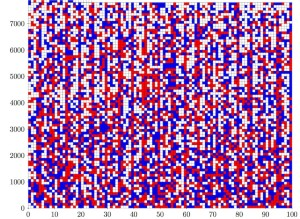The University of Texas’s Stampede supercomputer, on which the 200-terabyte maths proof was solved. Credit: University of Texas

Three computer scientists have announced the largest-ever mathematics proof: a file that comes in at a whopping 200 terabytes1, roughly equivalent to all the digitized text held by the US Library of Congress. The researchers have created a 68-gigabyte compressed version of their solution — which would allow anyone with about 30,000 hours of spare processor time to download, reconstruct and verify it — but a human could never hope to read through it.

Computer-assisted proofs too large to be directly verifiable by humans have become commonplace, and mathematicians are familiar with computers that solve problems in combinatorics — the study of finite discrete structures — by checking through umpteen individual cases. Still, “200 terabytes is unbelievable”, says Ronald Graham, a mathematician at the University of California, San Diego. The previous record-holder is thought to be a 13-gigabyte proof2, published in 2014.

The puzzle that required the 200-terabyte proof, called the Boolean Pythagorean triples problem, has eluded mathematicians for decades. In the 1980s, Graham offered a prize of US\$100 for anyone who could solve it. (He duly presented the cheque to one of the three computer scientists, Marijn Heule of the University of Texas at Austin, earlier this month.) The problem asks whether it is possible to colour each positive integer either red or blue, so that no trio of integers a, b and c that satisfy Pythagoras’ famous equation a2 + b2 = c2 are all the same colour. For example, for the Pythagorean triple 3, 4 and 5, if 3 and 5 were coloured blue, 4 would have to be red.

In a paper posted on the arXiv server on 3 May, Heule, Oliver Kullmann of Swansea University, UK, and Victor Marek of the University of Kentucky in Lexington have now shown that there are many allowable ways to colour the integers up to 7,824 — but when you reach 7,825, it is impossible for every Pythagorean triple to be multicoloured1. There are more than 102,300 ways to colour the integers up to 7,825, but the researchers took advantage of symmetries and several techniques from number theory to reduce the total number of possibilities that the computer had to check to just under 1 trillion. It took the team about 2 days running 800 processors in parallel on the University of Texas’s Stampede supercomputer to zip through all the possibilities. The researchers then verified the proof using another computer program.The numbers 1 to 7,824 can be coloured either red or blue so that no trio a, b and c that satisfies a2 +b2 = c2 is all the same colour. The grid of 7,824 squares here shows one such solution, with numbers coloured red or blue (a white square can be either). But for the numbers 1 to 7,825, there is no solution. Credit: Marijn Heule

Facts vs theory

The Pythagorean triples problem is one of many similar questions in Ramsey theory, an area of mathematics that is concerned with finding structures that must appear in sufficiently large sets. For example, the researchers think that if the problem had allowed three colours, rather than two, they would still hit a point where it would be impossible to avoid creating a Pythagorean triple where a, b and c were all the same colour; indeed, they conjecture that this is the case for any finite choice of colours. Any proof for more colours will probably be much larger even than the 200-terabyte 2-colour proof, unless researchers can simplify the case-by-case checking process with a breakthrough in understanding.

Although the computer solution has cracked the Boolean Pythagorean triples problem, it hasn’t provided an underlying reason why the colouring is impossible, or explored whether the number 7,825 is meaningful, says Kullmann. That echoes a common philosophical objection to the value of computer-assisted proofs: they may be correct, but are they really mathematics? If mathematicians’ work is understood to be a quest to increase human understanding of mathematics, rather than to accumulate an ever-larger collection of facts, a solution that rests on theory seems superior to a computer ticking off possibilities.

That did ultimately occur in the case of the 13-gigabyte proof from 2014, which solved a special case of a question called the Erdős discrepancy problem. A year later, mathematician Terence Tao of the University of California, Los Angeles, solved the general problem the old-fashioned way3 — a much more satisfying resolution.Credit: Marijn Heule